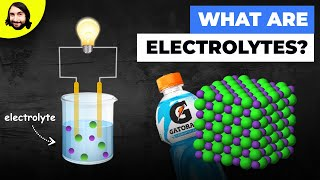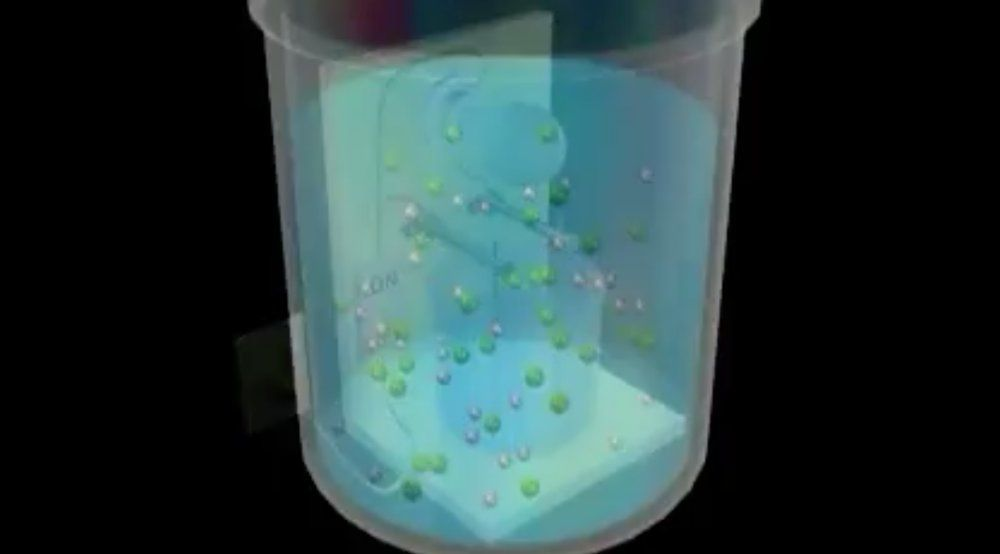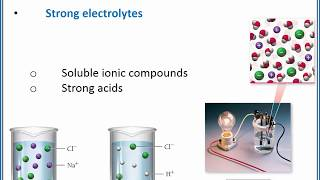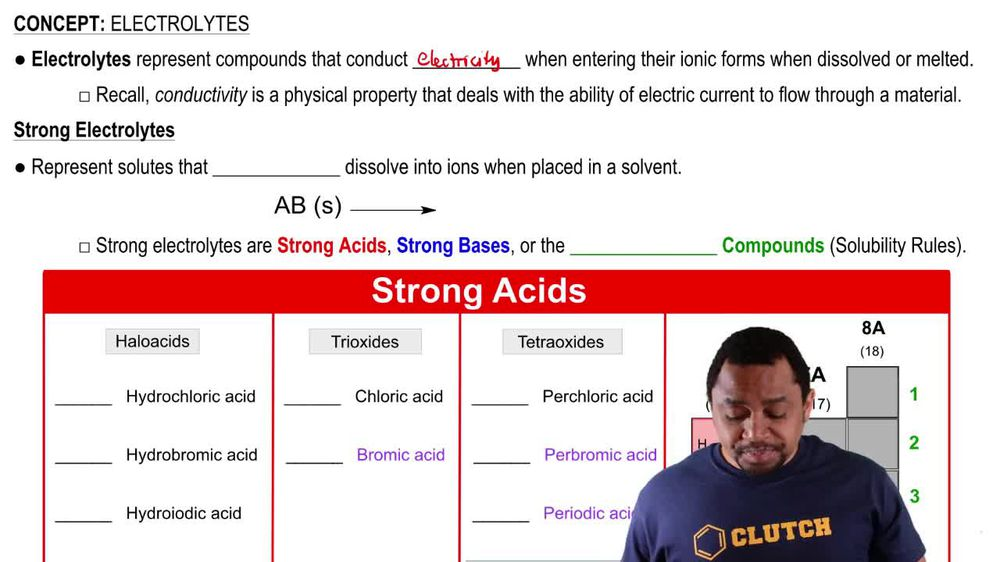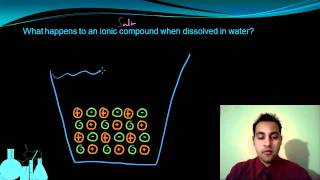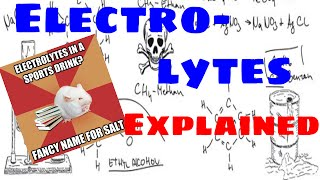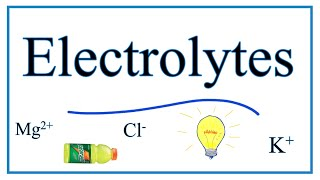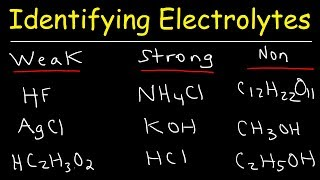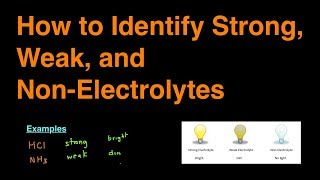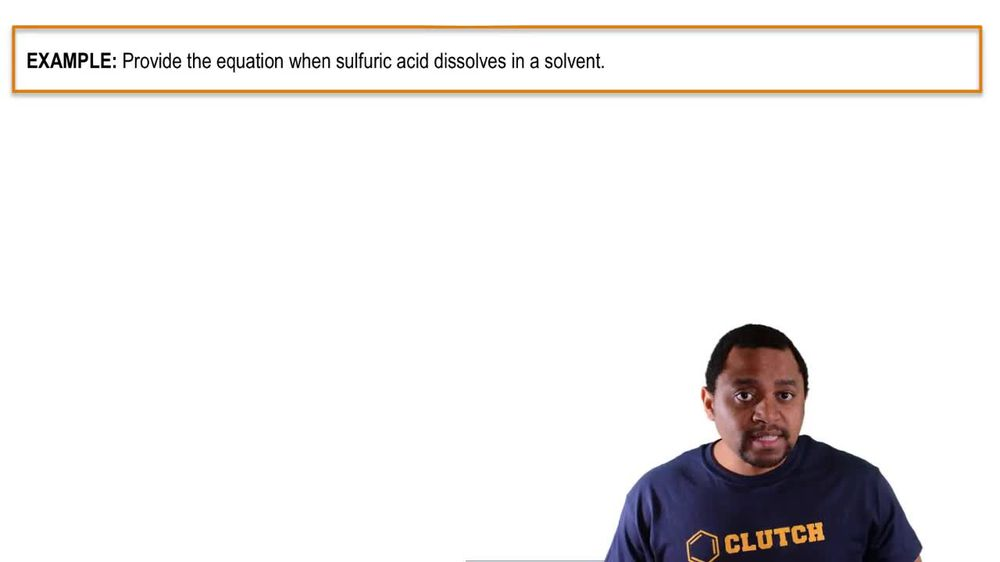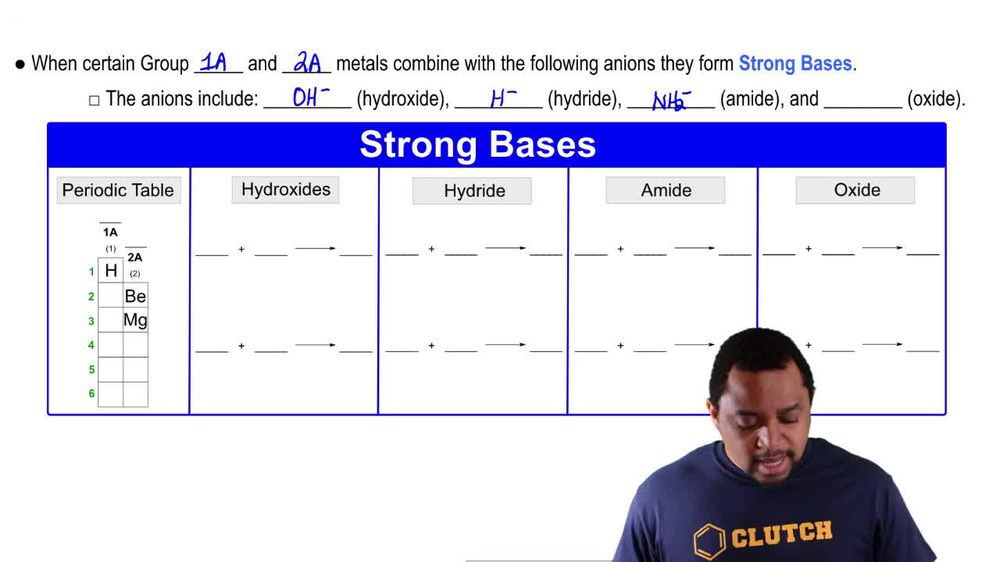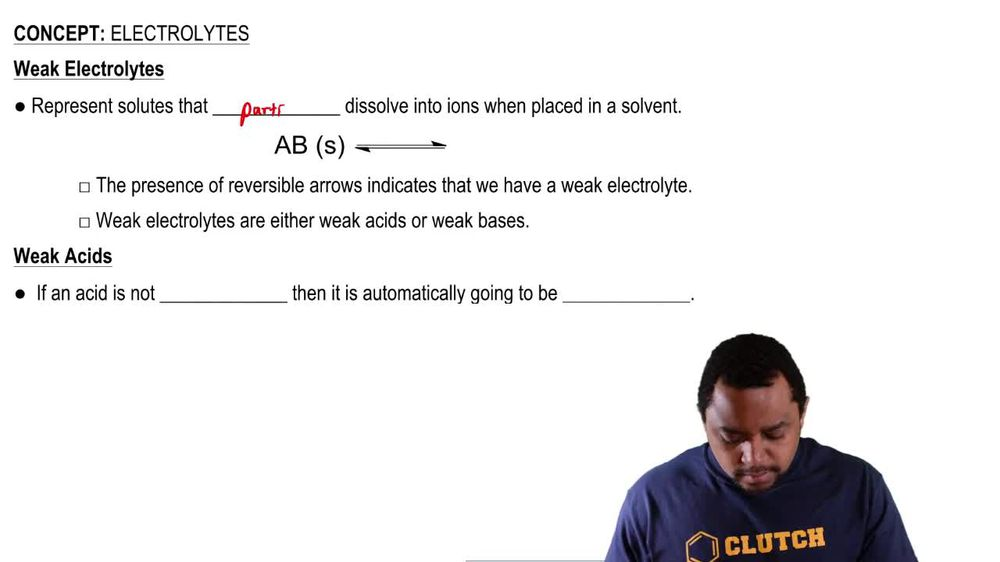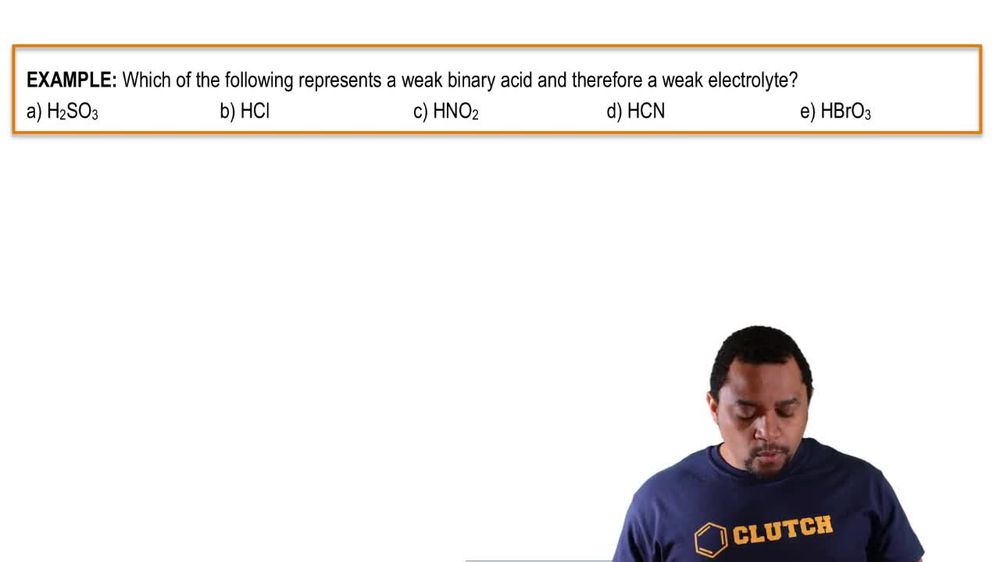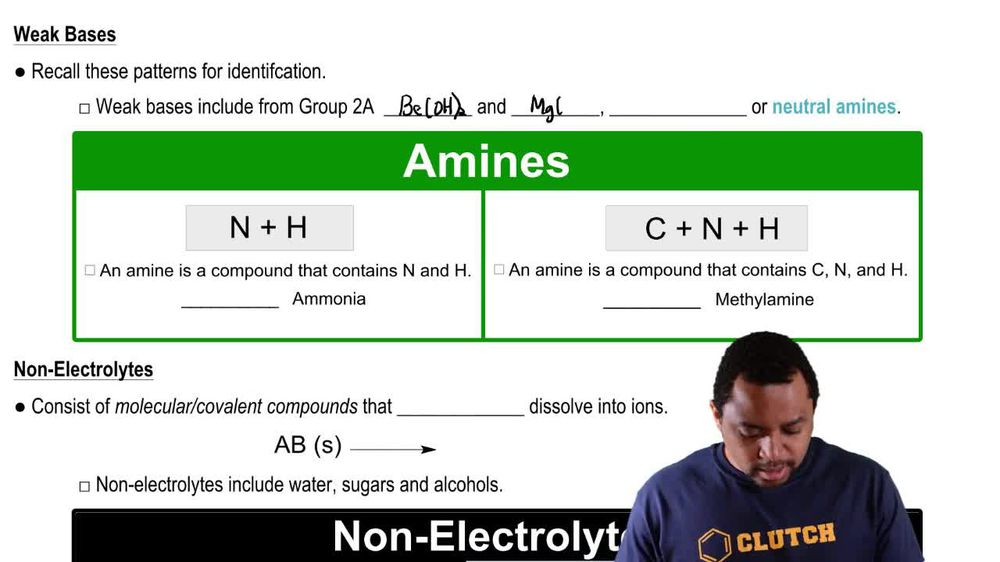Start typing, then use the up and down arrows to select an option from the list.
1. 6. Chemical Quantities & Aqueous Reactions2. Electrolytes# Electrolytes Concept 2

by Jules Bruno
99 views
1
1
here. We're going to say when a group one A and to a metals combined with the following an ions, they form strong bases. So the and ions include hydroxide ion which is O h minus hydride ion, which is h minus the, um I'd ion which is N h two minus and the oxide ion, which is o to minus the group one and two medals include we're gonna have here lithium sodium, potassium, rubidium and cesium. And then in group two way, we're gonna have calcium, strontium and barium. So it's just the ones that I have written in which will be the medals when they combined with these four negative ions will create a strong base. So here for hydroxide. So let's say I took lithium ion and hydroxide ion and then I took calcium ion and hydroxide ion. So remember, with charges, when the numbers are the same, they cancel out. So this would just be ally Ohh! Lithium hydroxide Here the numbers are different. There's a two here in a one here. So too would come here and one would come here. That, too, is for all of the calcium hydroxide, all of the hydroxide ions are the B C A O. H two Hi dry. So let's say I took an A plus and H minus here. The numbers are the same. So they just can't slot to give me an H, which is sodium hydride here. Let's say we had S r two plus and H minus one. So again, the numbers are different. When they're different, they crisscross. So to from here would come here and one from here would come here to give me strong to him hydride. Then we have a mind ion. So let's say we did potassium with NH two minus. That would give me potassium of mind here if we have barium with the, um I'd ion The numbers are different. So too would come here and one would come here. So this would be berry. Um um I'd so be a NH two parentheses, too. And then we have oxide. So let's say we did see us plus one in o to minus. The numbers are different. Soul. What happens? They crisscrossed One comes here to come here. So this would be see us 20 C. S c um oxide. And then let's say we had see a two plus and O to minus here. The numbers are the same soul. They don't crisscross, they just cancel out. So calcium oxide. So here we're just looking at different combinations off the medals that I have from groups one A and a.m. blue mixed with one of these negative ions. Doing that will form a strong base. Remember, As a result of being a strong base, you are a strong electrolyte.